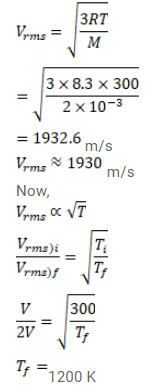# Use R=8.3 J/mol-K wherever required. Find the rms speed of hydrogen moleculesQuestion:

Use $\mathrm{R}=8.3 \mathrm{~J} / \mathrm{mol}-\mathrm{K}$ wherever required.

Find the rms speed of hydrogen molecules in a sample of hydrogen gas at $300 \mathrm{~K}$. Find the temperature at which the rms speed is double the speed calculated in the previous part.

Solution: